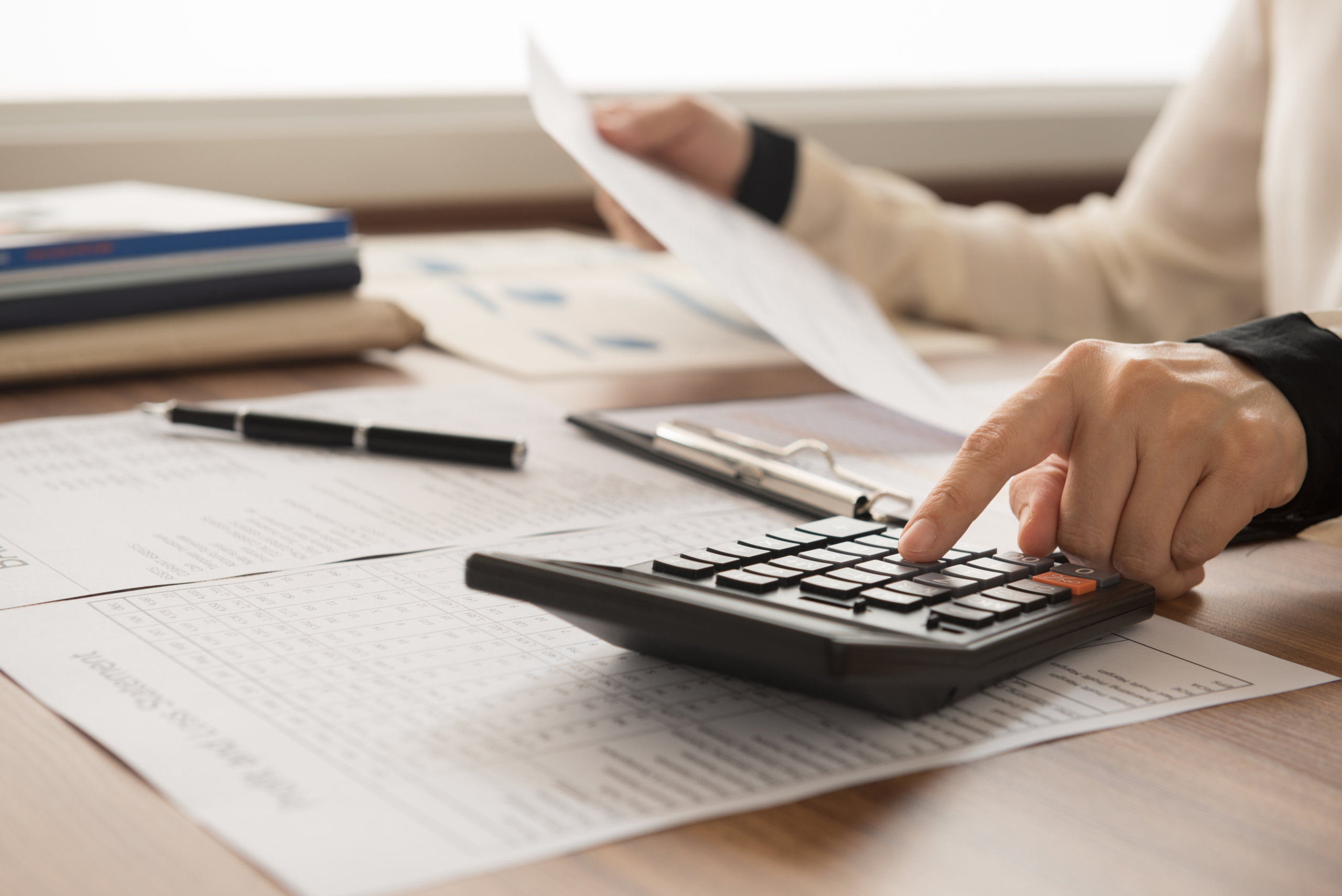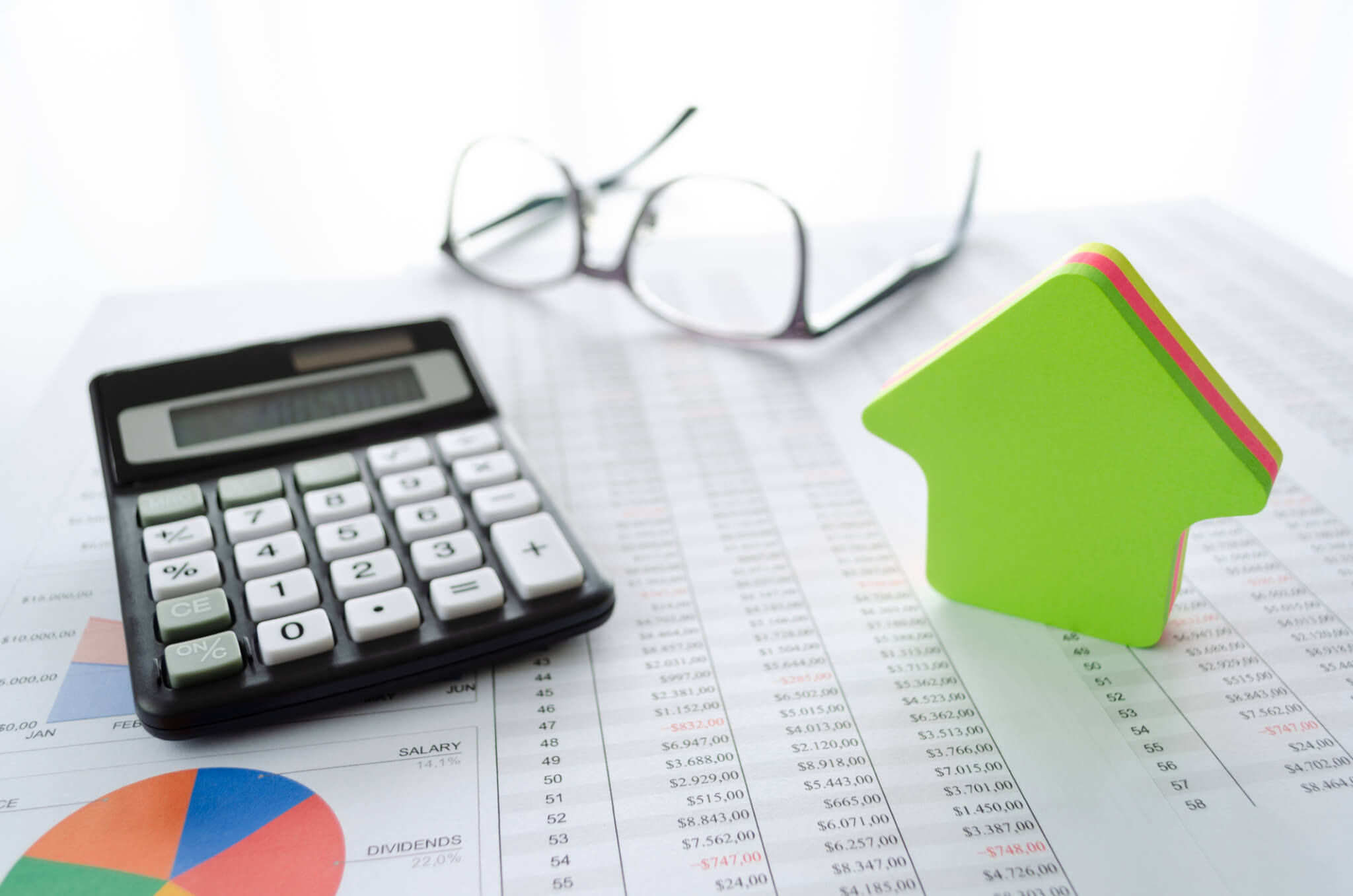# Cap Rate vs Cash on Cash: Two Magic Metrics for Real Estate Investorsby Jeff Rohde, posted in Finances

Cap rate and cash on cash return are two important metrics that real estate investors use to analyze rental property, but each calculation is used for different reasons.

In this article, we’ll explain how cap rate and cash on cash return calculations work, and why investors should use both metrics when analyzing potential investment property.

## Is Cap Rate The Same as Cash on Cash?

There are three significant differences between the cap rate and cash on cash return calculations:

### Financing Costs

Mortgage loan costs are excluded from the cap rate calculation but included in the cash on cash return. By not including financing costs in the cap rate, investors can make a better apples-to-apples comparison of similar properties in the same area, because how a property is financed and the amount of leverage used varies from one investor to the next.

Financing costs are included when calculating cash on cash return, to measure how much profit is received for each dollar invested. For example, some investors prefer to use a conservative LTV of 75%, while other buyers with a short-term hold (such as fix-and-flippers) put down as little money as possible.

### All Cash Scenario

For investors who pay for a property all in cash, the cap rate and cash on cash return results are the same. Another way of thinking about cash on cash return is that the formula assumes you are obtaining a loan to purchase the home, by following the principle of OPM (other people’s money).

### Equation Itself

Lastly, the denominator used in the cap rate and cash on cash return formulas is different. In the cap rate calculation, the bottom number is the purchase price or market value. With the cash on cash return formula, the bottom number is the amount of cash invested, such as the property down payment.

Now let’s look at how real estate investors calculate and use cap rate and cash on cash return.## How to Calculate Cap Rate

Cap rate (short for capitalization rate) measures the return or profitability of similar assets in the same market, submarket, or neighborhood. It’s important to understand that cap rates for the same type of property vary from market to market, due to factors such as supply and demand, and the general cost of living.For example, some single-family homes in California listed for sale to investors on the Roofstock Marketplace have cap rates of less than 3%. On the other hand, many single-family rental houses for sale in a lower cost of living state such as Alabama offer investors cap rates of 6% or more.

### Cap Rate Formula and Calculation

The cap rate formula looks like this:

• Cap Rate = NOI / Market Value

NOI is the property’s net operating income excluding any financing costs such as a mortgage payment. Market value is the current value of the property, or the price the property was purchased at if the investment is brand new.

You can also use the cap rate formula to calculate what the NOI should be and what the market value should be, provided you have two of the three variables. Let’s assume a single-family home has a market value of \$150,000 with an NOI of \$10,500.

The cap rate is:

• \$10,500 NOI / \$150,000 Market Value = .07 or 7% Cap Rate

If similar properties in the same market have a cap rate of 6.5%, a home with an asking price of \$150,000 should generate an NOI of:

• \$150,000 Market Value x 6.5% Cap Rate = \$9,750 NOI

Or, if cap rates for similar property in the same market are 7.5% and the NOI for a property you are thinking about investing in is \$11,000, the market value should be:

• \$11,000 NOI / 7.5% Cap Rate = \$146,667 Market Value

### What is a Good Cap Rate?

As a rule of thumb, the property generating the highest cap rate may be the better investment for a buyer, because the potential profit is higher, everything else being equal.

However, if a property has a higher (or lower) cap rate than comparable properties, an investor should always ask why.

A property that has a lot of deferred maintenance may have a lower asking price because of the extra money a new owner will have to put in. Or, the current market value of the property may be higher because the seller is collecting above-market rents.## How to Calculate Cash on Cash

Cash on cash return provides investors with a more specific indication of potential financial performance based on the amount of leverage being used. The cash on cash return formula measures the net cash flow as a percentage of the total amount of cash invested.

Unlike the cap rate formula which should only be used to compare similar properties in the same market, the cash on cash return formula can be used to compare potential cash returns between properties in different real estate markets.

### Cash on Cash Return Formula and Calculation

The cash on cash return formula looks like this:

• Cash on Cash Return = Annual Cash Flow (after debt service) / Total Cash Invested

Let’s assume you purchase a \$150,000 single-family rental home with a conservative down payment of 25%. The total cash invested is \$37,500 (\$150,000 x 25% down payment). By deducting annual mortgage payments (P&I) of \$5,675 from the NOI of \$10,500, your annual cash flow is \$4,824.

The cash on cash return is:

• \$4,824 Annual Cash Flow / \$25,000 Total Cash Invested = 19.3%

If you pay all cash for the property, your cash on cash return is the same as your cap rate, because there are no mortgage payments that reduce your cash flow:

• \$10,500 Annual Cash Flow / \$150,000 Total Cash Invested = 7%

Or, if you could use a lower down payment of 10%. In this case, the total cash invested is \$15,000 and your annual cash flow after the mortgage payments is \$3,696 (\$10,500 NOI – \$6,804 mortgage payments P&I):

• \$3,696 Annual Cash Flow / \$15,000 Total Cash Invested = 24.6%

### What is a Good Cash on Cash Return?

Comparing the return you would receive on your cash if you invested it somewhere else is a good way to think about what good cash on cash return is.

For example, a “risk-free” investment like a 10-year Treasury currently yields about 1.7%. On the other hand, the High Yield Bond ETF (HYLD) has an annual dividend yield of about 7.3% (May 2021).

That makes the cash on cash return on real estate look pretty good, especially given all of the additional tax benefits real estate receives, such as depreciation expense to reduce taxable net income.## Comparing Cap Rate to Cash on Cash

Now let’s compare the cap rate and cash on cash return of a single-family rental home.

For this example, the house has a market value of \$125,000, gross monthly rental income of \$1,200, and operating expenses of \$600. Financing the property with a 25% down payment (\$31,250) results in a monthly mortgage payment of \$394 (P&I).

By the way, property taxes and insurance are not included as part of the mortgage payment because they are treated as normal operating expenses.

Before we can compare the cap rate to the cash on cash return, we need to do two calculations. First, we need to calculate the annual NOI. We do that by subtracting the monthly operating expenses from the gross monthly rental income, then multiplying by 12 months:

• \$1,200 monthly rental income – \$600 monthly operating expenses = \$600 x 12 months = \$7,200 NOI

Then, we need to calculate the cash flow after debt service. We do that by subtracting the annual mortgage payment from the annual NOI:

• \$394 monthly mortgage payment x 12 months = \$4,728 annual mortgage payment
• \$7,200 annual NOI – \$4,728 annual mortgage payment = \$2,472 annual cash flow

With this information in hand, we’re ready to calculate the cap rate and cash on cash return for the single-family rental property.

Cap Rate

• Cap Rate = NOI / Market Value
• \$7,200 NOI / \$125,000 Market Value = 5.76% Cap Rate

Cap rates for single-family rental homes in the neighborhood are closer to 6%, which means a buyer may want to make an offer for \$120,000 to avoid overpaying for the house.

Cash on Cash Return

• Cash on Cash Return = Annual Cash Flow / Total Cash Invested
• \$2,472 Annual Cash Flow / \$31,250 Total Cash Invested  = 7.9%

If we can buy the property for a fair market value of \$120,000, the annual mortgage expense would decrease to \$4,536 and the annual cash flow would increase to \$2,664, resulting in a cash on cash return of:

• \$2,664 Annual Cash Flow / \$30,000 Total Cash Invested = 8.9%## Is Cap Rate Better Than Cash on Cash?

When you analyze rental property for potential investment, both cap rate and cash on cash return calculations should be used. Cap rate is a good way to find prospective deals that make sense. If the cap rate in the market is 6%, an investor might decide to screen out properties with cap rates below 6%.

Once an investor has several properties to choose from, cash on cash return is a good way to select the investment generating the highest return for the cash invested. When comparing the cash on cash returns from alternative choices, it’s important to use the same financing assumptions for each analysis.

## Other Financial Formulas to Know

In addition to cap rate and cash on cash return, other financial formulas and metrics that rental property investors use include:

ROI (return on investment)

• Gain on Investment – Cost of Investment / Cost of Investment
• \$175,000 Gain on Investment – \$125,000 Cost of Investment / \$125,000 Cost of Investment = 40% ROI

IRR (internal rate of return)

Rent/Cost

• Monthly Rent / Property Price
• \$1,200 Monthly Rent / \$125,000 Property Price = 1% which is the minimum that most investors look for

DSCR (debt service coverage ratio)

• DSCR = NOI / Annual Debt Service
• \$7,200 NOI / \$4,536 Annual Debt Service = 1.59 DSCR which is above the 1.2 ratio that many lenders like to see

### Final Thoughts on This Topic

Two of the most commonly used metrics that real estate investors used to analyze the potential returns from rental property are cap rate and cash on cash return.

Cap rate measures the potential profit from an investment without factoring in financing.

Cash on cash return tells you how much profit you receive for each dollar invested.

Rental property investors use both calculations to determine the best potential real estate investments.